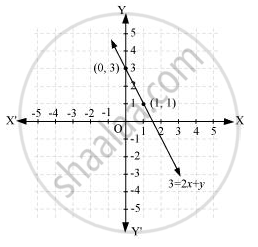# Draw the Graph of the Following Linear Equations in Two Variables:- 3 = 2x + y - Mathematics

Draw the graph of the following linear equations in two variables:- 3 = 2x + y

#### Solution

It can be observed that x = 0, y = 3 and x = 1, y = 1 are solutions of the above equation. Therefore, the solution table is as follows.

 x 0 1 y 3 1

The graph of this equation is constructed as follows.Concept: Graph of a Linear Equation in Two Variables
Is there an error in this question or solution?

#### APPEARS IN

NCERT Class 9 Maths
Chapter 4 Linear Equations in two Variables
Exercise 4.3 | Q 1.4 | Page 74